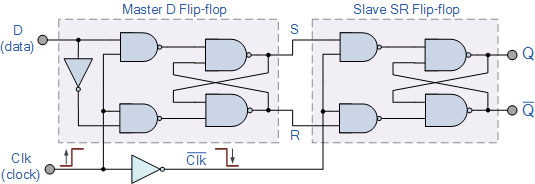# The D-type Flip Flop

## The D-type Flip Flop

One of the main disadvantages of the basic SR NAND Gate bistable circuit is that the indeterminate input condition of “SET” = logic “0” and “RESET” = logic “0” is forbidden. This state will force both outputs to be at logic “1”, over-riding the feedback latching action and whichever input goes to logic level “1” first will lose control, while the other input still at logic “0” controls the resulting state of the latch.But in order to prevent this from happening an inverter can be connected between the “SET” and the “RESET” inputs to produce another type of flip flop circuit known as a Data Latch, Delay flip flop, D-type Bistable, D-type Flip Flop or just simply a D Flip Flop as it is more generally called.

The D Flip Flop is by far the most important of the clocked flip-flops as it ensures that ensures that inputs S and R are never equal to one at the same time. The D-type flip flop are constructed from a gated SR flip-flop with an inverter added between the S and the R inputs to allow for a single D (data) input.

Then this single data input, labelled D, is used in place of the “set” signal, and the inverter is used to generate the complementary “reset” input thereby making a level-sensitive D-type flip-flop from a level-sensitive RS-latch as now S = D and R = not D as shown.

### D-type Flip-Flop CircuitWe remember that a simple SR flip-flop requires two inputs, one to “SET” the output and one to “RESET” the output. By connecting an inverter (NOT gate) to the SR flip-flop we can “SET” and “RESET” the flip-flop using just one input as now the two input signals are complements of each other. This complement avoids the ambiguity inherent in the SR latch when both inputs are LOW, since that state is no longer possible.

Thus this single input is called the “DATA” input. If this data input is held HIGH the flip flop would be “SET” and when it is LOW the flip flop would change and become “RESET”. However, this would be rather pointless since the output of the flip flop would always change on every pulse applied to this data input.

To avoid this an additional input called the “CLOCK” or “ENABLE” input is used to isolate the data input from the flip flop’s latching circuitry after the desired data has been stored. The effect is that D input condition is only copied to the output Q when the clock input is active. This then forms the basis of another sequential device called a D Flip Flop.

The “D flip flop” will store and output whatever logic level is applied to its data terminal so long as the clock input is HIGH. Once the clock input goes LOW the “set” and “reset” inputs of the flip-flop are both held at logic level “1” so it will not change state and store whatever data was present on its output before the clock transition occurred. In other words the output is “latched” at either logic “0” or logic “1”.

### Truth Table for the D-type Flip Flop

 Clk D Q Q Description ↓ » 0 X Q Q Memory no change ↑ » 1 0 0 1 Reset Q » 0 ↑ » 1 1 1 0 Set Q » 1

Note that: and indicates direction of clock pulse as it is assumed D-type flip flops are edge triggered

## The Master-Slave D Flip Flop

The basic D-type flip flop can be improved further by adding a second SR flip-flop to its output that is activated on the complementary clock signal to produce a “Master-Slave D-type flip flop”. On the leading edge of the clock signal (LOW-to-HIGH) the first stage, the “master” latches the input condition at D, while the output stage is deactivated.